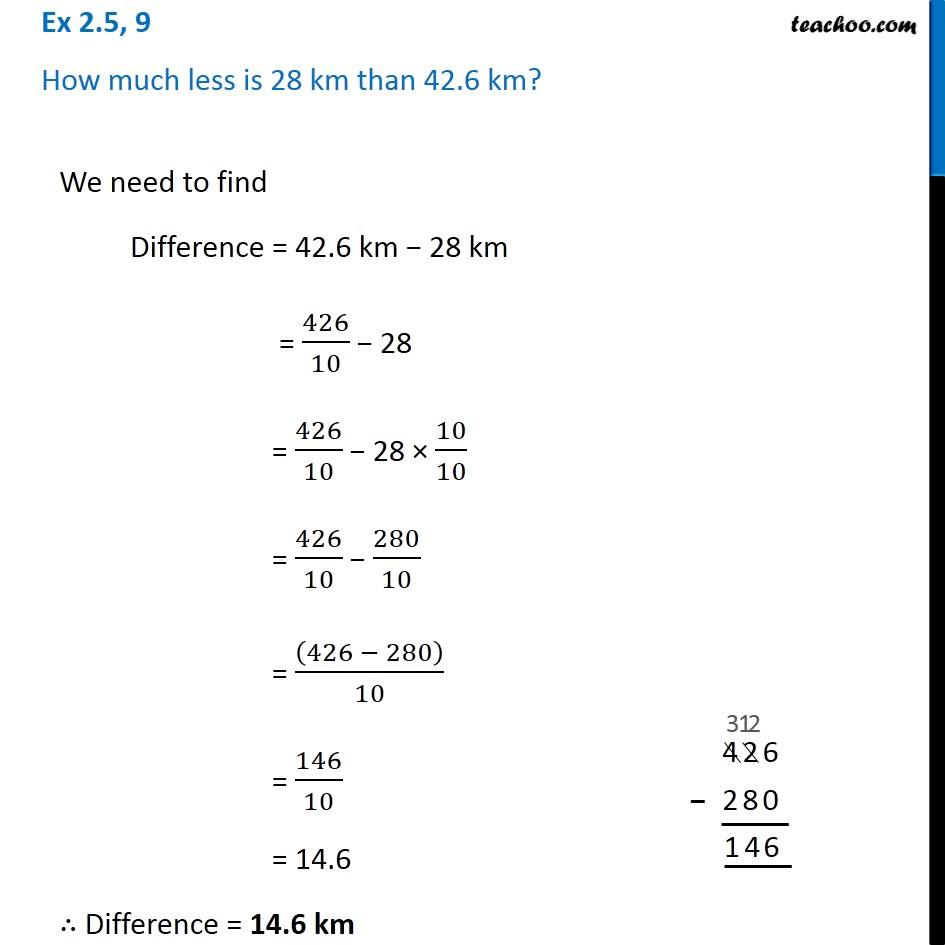Ex 2.5

Chapter 2 Class 7 Fractions and Decimals
Serial order wise### Transcript

Ex 2.5, 9 How much less is 28 km than 42.6 km?We need to find Difference = 42.6 km − 28 km = 426/10 − 28 = 426/10 − 28 × 10/10 = 426/10 − 280/10 = ((426 − 280))/10 = 146/10 = 14.6 ∴ Difference = 14.6 km